# Oberseminar Analysis Summer 2015

## Organizers: B. Zwicknagl, S. Conti, H. Koch, S. Müller, B. Niethammer, M. Rumpf, C. Thiele, J. López-Velázquez

• Thursday, April 2, 6:10 p.m., SR 0.011
Videoseminar Berkeley/Bonn/Paris-Nord/Zurich
Piotr Bizoń (Jagiellonian University, Krakow)
Blowup for supercritical equivariant wave maps
We consider equivariant wave maps from R^{d+1} into S^d for d\geq 3. Using mixed analytic and numerical tools we describe the dynamics of generic blowup and the threshold for blowup. We hope that our plausibility arguments will stimulate rigorous studies of this problem. This is joint work with Pawel Biernat and Maciej Maliborski.

• Thursday, May 7, 2:15 p.m., Lipschitz-Saal
Ian Tobasco (Courant Institute, New York)
Energy scaling laws for an axially compressed thin elastic cylinder
A longstanding open problem in elasticity is to identify the minimum energy scaling law of a crumpled sheet of paper whose thickness tends to zero. Though much is known about scaling laws for thin sheets in tensile settings, the compressive regime is mostly unexplored. I will discuss the case of an axially confined thin elastic cylinder which is prevented from inward displacement by a hard mandrel core. My focus in this talk will be the dependence of the minimum energy on the thickness of the cylinder in the Foppl-von Karman model. I will prove upper and lower bounds for this scaling.

• Friday, May 15, 2:15 p.m., SR 0.011
Annegret Burtscher

Geodesic incompleteness in general relativity

The general theory of relativity describes the effect of gravitation in

terms of the geometry of spacetimes via the Einstein equations. In the

1950s the initial value formulation and local existence of solutions to

the Einstein equations were established. As of yet the global structure of

solutions is much less understood, in general, singularities seem

unavoidable. The Penrose singularity theorems give some glimpse of this

singular nature by relating geodesic incompleteness to the existence of

trapped surfaces. In this talk we will see how such trapped surfaces can

form during evolution from regular initial data, illustrated for

spherically symmetric solutions of the Einstein-Euler equations.

• Thursday, May 21, 2:15 p.m., SR 0.011
Barbora Benesova (Wuerzburg University)
Non-interpenetration conditions in elasticity
In this talk we characterize necessary and sufficient conditions on the stored energy density in order to assure weak* lower semicontinuity on the set of quasiconformal as well as bi-Lipschitz functions in the plane. This problem is motivated by variational problems in nonlinear elasticity where the orientation preservation and injectivity of the admissible deformations are key requirements. Generally speaking, the main difficulty in finding such conditions is that any set of injective functions is non-convex. Thus, standard cut-off techniques that modify the generating sequence to have the same boundary conditions as the limit generally fail; however, the standard proofs of in calculus of variations rely on such methods. We obtain this cut-off by modifying the generating sequence (on a set of gradually vanishing measure near the boundary) first on a one dimensional grid and then rely on extension theorems. If time permits, we will also briefly touch the topic of non-interpenetration in thin films. Contrary to the bulk, here injectivity is not equivalent to non-interpenetration and we will discuss a possible definition of non-interpenetration in this setting. This is joint work with Martin Kruzik (Prague) and Malte Kampschulte (Aachen).

• Thursday, June 25, 2:15 p.m., Lipschitz-Saal
Birgit Schörkhuber (University of Vienna)
Blowup in semilinear wave equations
In this talk I will consider wave equations with focusing power type
nonlinearities $$f(u)= u|u|^{(p-1)}$$. It is well known that for such models
finite time blowup of solutions from smooth, compactly supported initial
data can occur. I discuss recent progress in the investigation of stable
blowup dynamics for radial equations in odd space dimensions $$d \geq 3$$. The
focus will be on superconformal nonlinearities $$p > (d+3)/(d-1)$$.

• Thursday, July 2, 2:15 p.m., SR 0.011
Jeremy L. Marzuola (University of North Carolina, Chapel Hill)
Spectral Cluster estimates on polygonal domains
In joint work with Matt Blair and Austin Ford, we look at the $$L^p$$ bounds on eigenfunctions for polygonal domains (or more generally Euclidean surfaces with conic singularities) by analysis of the wave operator on the flat Euclidean cone.  Using explicit oscillatory integrals and relying on the fundamental solution to the wave equation in geometric regions related to flat wave propagation and diffraction by the cone point, we can prove spectral cluster estimates equivalent to those in works on smooth Riemannian manifolds.

• Thursday, July 9, 2:15 p.m., Lipschitz-Saal
Henrik Schumacher (Georg-August Universität Göttingen)
Variational Convergence of Discrete Minimal Surfaces
A straightforward discretization of the least area problem for surfaces of fixed topological type under Dirichlet boundary conditions is by minimizing the area among triangle meshes with fixed combinatorics, spanned into a boundary of closed polygonal lines. We show Kuratowski convergence (under refinement) of discrete $\delta$-minimizers to smooth minimizers if both the smooth and the discrete boundary value problems are solvable and if the solutions fulfill certain a priori assumptions (e.g., a uniform bound in the $W^{2,\infty}$-norm for minimizers of the smooth problem). Therefore, we develop a shape space of unparameterized Lipschitz immersions, topologized by a certain metric. Apart from the problem of existence, the approach is independent of the genus, the orientability and the number of boundary components of the considered surfaces.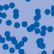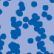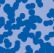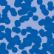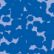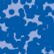## News

Florian Schweiger erhielt den Hausdorff-Gedächtnispreis 2021 der Fachgruppe Mathematik für die beste Dissertation. Er fertigte die Dissertation unter der Betreuung von Prof. Stefan Müller an. Unter anderen wurde Vanessa Ryborz mit einem Preis der Bonner Mathematischen Gesellschaft für ihre von Prof. Sergio Conti betreute Bachelorarbeit ausgezeichnet. (18.01.2022)

Prof. Dr. Sergio Albeverio has been elected into the Academia Europaea and the Accademia Nazionale dei Lincei (more; 02.12.2021).

Der SFB 1060 Die Mathematik der emergenten Effekte hat eine dritte Förderperiode erhalten. (26.11.20)

Prof. Dr. Andreas Eberle erhält den diesjährigen Lehrpreis der Universität Bonn. (22.07.2020)

Herr Dr. Richard Höfer erhielt den Hausdorff-Gedächtnispreis 2019 der Fachgruppe Mathematik für die beste Disseration. Betreut wurde die Arbeit von Prof. J. Velázquez (29.01.2020).

Contact

Managing Director: Prof. Dr. Massimiliano Gubinelli